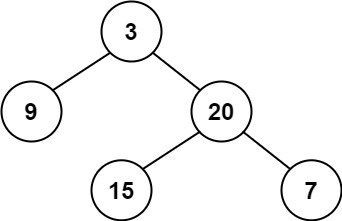# Balanced Binary Tree

## 1: U-nderstand

Understand what the interviewer is asking for by using test cases and questions about the problem.

• Established a set (2-3) of test cases to verify their own solution later.
• Established a set (1-2) of edge cases to verify their solution handles complexities.
• Have fully understood the problem and have no clarifying questions.
• Have you verified any Time/Space Constraints for this problem?
• What is the type of tree we are working with?
• The input tree will be a binary tree
• Can I assume the tree will be complete?
• No. A node may have less than 2 children
• Can I expect to receive an empty tree as input?
• Yes, the input tree can have 1-10000 nodes.
• What is the time and space constraints for this problem?
• `O(n)` time complexity and `O(1)` excluding the recursion call stack.
``````HAPPY CASE
Input: root = [3,9,20,null,null,15,7]
Output: true````````````Input: root = [1,2,2,3,3,null,null,4,4]
Output: false````````````EDGE CASE
Input: root = []
Output: true``````

## 2: M-atch

Match what this problem looks like to known categories of problems, e.g. Linked List or Dynamic Programming, and strategies or patterns in those categories.

If you are dealing with Binary Trees some common techniques you can employ to help you solve the problem:

• Think about appropriate Tree Traversal: Pre-Order, In-Order, Post-Order, Level-Order
• Since we need to get the height of the tree, we will need to traverse all nodes down the tree, we should recursively return depth of each node upward to parent. a Pre-Order traversal would be appropriate
• Store nodes within a HashMap to refer to later
• We don't need to access the nodes or their values after they have been processed, so this technique is not useful for this problem
• Using Binary Search to find an element
• We are not looking to find an element in this problem so this technique is not useful for this problem
• Applying a level-order traversal with a queue
• Since we need to find the height of the tree, we could get nodes by level. However, we need to do so for each node to check the balance at each node. a level-order traversal using a queue would not be appropriate

## 3: P-lan

Plan the solution with appropriate visualizations and pseudocode.

General Idea: Recursively ask for child height in tree and add one for parent. Check the balance at each node by looking at the right and left height of tree.

``````1. Create function to return the height of tree and collect balance at each node
a. Basecase is a Null Node, return 0
b. Collect information regarding balance of node
c. Recursively return the max between height of left node and right node and add one for current node.
2. Create variable to hold balance information
3. Call the function to check balance of each node
4. Return the balance``````

⚠️ Common Mistakes

• Choosing the wrong traversal type
• Try to walk through the problem by hand and see the order in which you are processing the nodes. This will clue you into the type of traversal necessary

## 4: I-mplement

Implement the code to solve the algorithm.

``````# Definition for a binary tree node.
# class TreeNode:
#     def __init__(self, val=0, left=None, right=None):
#         self.val = val
#         self.left = left
#         self.right = right
class Solution:
def isBalanced(self, root: Optional[TreeNode]) -> bool:
# Create function to return the height of tree and collect balance at each node
def helper(root: Optional[TreeNode]) -> int:
# Basecase is a Null Node, return 0
if not root:
return 0

# Collect information regarding balance of node
leftHeight = helper(root.left)
rightHeight = helper(root.right)
if abs(rightHeight - leftHeight) > 1:
nonlocal balanced
balanced = False
return 0

# Recursively return the max between height of left node and right node and add one for current node.
return max(leftHeight, rightHeight) + 1

# Create variable to hold balance information
balanced = True

# Call the function to check balance of each node
helper(root)

# Return the balance
return balanced``````
``````class Solution {
public boolean isBalanced(TreeNode root) {
// If the tree is empty, we can say it’s balanced...
if (root == null)  return true;
// Call the function to check balance of each node and return the balance
if (Height(root) == -1)  return false;
return true;
}
// Create function to return the height of tree and collect balance at each node
public int Height(TreeNode root) {
// Basecase is a Null Node, return 0
if (root == null)  return 0;

// Collect information regarding balance of node
int leftHeight = Height(root.left);
int rightHight = Height(root.right);

// Recursively return the max between height of left node and right node and add one for current node.
// In case of left subtree or right subtree unbalanced, return -1...
if (leftHeight == -1 || rightHight == -1)  return -1;
// If their heights differ by more than ‘1’, return -1...
if (Math.abs(leftHeight - rightHight) > 1)  return -1;
// Otherwise, return the height of this subtree as max(leftHeight, rightHight) + 1...
return Math.max(leftHeight, rightHight) + 1;
}
}``````

## 5: R-eview

Review the code by running specific example(s) and recording values (watchlist) of your code's variables along the way.

• Trace through your code with an input to check for the expected output
• Catch possible edge cases and off-by-one errors

## 6: E-valuate

Evaluate the performance of your algorithm and state any strong/weak or future potential work.

Assume `N` represents the number of nodes in binary tree

• Time Complexity: `O(N)`, because we need to visit each node in the binary tree
• Space Complexity: `O(1)` because we exclude the recursive call stack. The recursive call stack may cost `O(N)` we need to account for unbalanced tree. In the general case `O(logN)` for balanced tree.